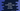# 6 different ways to sort an array in Kotlin## How to sort an array in Kotlin :

Sorting an array is really easy in Kotlin. Kotlin comes with different built-in functions to make it easier for us. In this tutorial, I will show you different ways to sort an array in Kotlin. You will learn how to sort a simple array and to sort an array of custom objects.

## Method 1: Sort an array in ascending order using sort() :

sort() function is used for ascending order sorting. sort() is defined as below :

``fun > Array.sort()``

It will sort the array in-place as its natural order of the elements. For example :

``````import java.util.*

fun main() {
val students = arrayOf(10,9,23,12,5,2,4,1)
println("Original array : \${Arrays.toString(students)}")

students.sort()
println("Sorted array : \${Arrays.toString(students)}")
}``````

It will print the below output :

``````Original array : [10, 9, 23, 12, 5, 2, 4, 1]
Sorted array : [1, 2, 4, 5, 9, 10, 12, 23]``````

Note that this function will modify the original array.

## Method 2: Sort an array in ascending order in a range :

We can use the sort(from,to) function to sort an array within a range. It takes two parameters: from index position and to index position. If you pass 0 and 5 respectively, it will only sort the elements starting from 0th position to 4th position, leaving other elements unchanged. The definition of this function is as below :

``public fun  Array.sort(fromIndex: Int = 0, toIndex: Int = size)``

### Example :

``````import java.util.*

fun main() {
val students = arrayOf(10,9,8,7,6,5,4,3,2,1)
println("Original array : \${Arrays.toString(students)}")

students.sort(0,5)
println("Sorted array from index 0 to 5 : \${Arrays.toString(students)}")
}``````

Output :

``````Original array : [10, 9, 8, 7, 6, 5, 4, 3, 2, 1]
Sorted array from index 0 to 5 : [6, 7, 8, 9, 10, 5, 4, 3, 2, 1]``````

As you can see, only the first 5 elements, i.e. elements from index 0 to index 4 are sorted in this example.

## Method 3: Sort an array in descending order :

sortDescending() is used to sort an array in descending order. Similar to sort(), it will sort the array in place. The syntax of this function is as below :

``public fun > Array.sortDescending()``

### Example :

``````import java.util.*

fun main() {
val students = arrayOf(1,5,7,8,2,3,9,10)
println("Original array : \${Arrays.toString(students)}")

students.sortDescending()
println("Sorted array : \${Arrays.toString(students)}")
}``````

Output :

``````Original array : [1, 5, 7, 8, 2, 3, 9, 10]
Sorted array : [10, 9, 8, 7, 5, 3, 2, 1]``````

Similar to sort(), we can also pass from and to index to this function to sort only a part of the array :

``````import java.util.*

fun main() {
val students = arrayOf(1,2,3,4,5,6,7,8,9,10)
println("Original array : \${Arrays.toString(students)}")

students.sortDescending(0, 5)
println("Sorted array from index 0 to 5 : \${Arrays.toString(students)}")
}``````

Output :

``````Original array : [1, 2, 3, 4, 5, 6, 7, 8, 9, 10]
Sorted array from index 0 to 5 : [5, 4, 3, 2, 1, 6, 7, 8, 9, 10]``````

## Method 4: Sort and return a new array :

sortedArray() and sortedArrayDescending functions are used to sort an array and return a new array instead of changing the original array.

``````fun main() {
val students = arrayOf(5,2,6,9,10,1,44,23)
println("Original array : \${students.contentToString()}")

val sortedArray = students.sortedArray()
println("Sorted array : \${sortedArray.contentToString()}")

val descendingSortedArray = students.sortedArrayDescending()
println("Sorted array in descending : \${descendingSortedArray.contentToString()}")
}``````

Output :

``````Original array : [5, 2, 6, 9, 10, 1, 44, 23]
Sorted array : [1, 2, 5, 6, 9, 10, 23, 44]
Sorted array in descending : [44, 23, 10, 9, 6, 5, 2, 1]``````

Note that the original array students remain the same in this example.

Instead of an Array, if you want a list to return, use sorted() and sortedDescending functions.

## Method 5: Sort an array of custom objects using a comparator :

Use sortWith function, that takes one comparator as a parameter to sort an array of custom objects.

``````fun main() {
val students = arrayOf(Student("Alex", 5), Student("Bob", 2), Student("Harry", 1))

println("Original array : ")
students.forEach { println(it) }

students.sortWith(Comparator { s1: Student, s2: Student -> s1.rank - s2.rank })

println("Final array : ")
students.forEach { println(it) }
}

data class Student(val name: String, val rank: Int)``````

Output :

``````Original array :
Student(name=Alex, rank=5)
Student(name=Bob, rank=2)
Student(name=Harry, rank=1)
Final array :
Student(name=Harry, rank=1)
Student(name=Bob, rank=2)
Student(name=Alex, rank=5)``````

As you can see, the original array is sorted as per the rank. Note that this function sorts the array in place.

• Use sortWith(comparator: Comparator,fromIndex: Int = 0,toIndex: Int = size) to sort an array within range.
• Use sortedArrayWith(comparator: Comparator) to return an array instead of sorting in-place.
• Use sortedWith(comparator: Comparator) to return a list.

## Method 6: Sort an array of custom objects using a selector function :

Instead of a comparator, we can also pass a selector function to the sortBy function for sorting an array. For example :

``````fun main() {
val students = arrayOf(Student("Alex", 5), Student("Bob", 2), Student("Harry", 1))

println("Original array : ")
students.forEach { println(it) }

students.sortBy { student -> student.rank}

println("Final array : ")
students.forEach { println(it) }
}

data class Student(val name: String, val rank: Int)``````

Output is :

``````Original array :
Student(name=Alex, rank=5)
Student(name=Bob, rank=2)
Student(name=Harry, rank=1)
Final array :
Student(name=Harry, rank=1)
Student(name=Bob, rank=2)
Student(name=Alex, rank=5)``````

This function also modifies the array in place.

• Use sortByDescending(crossinline selector: (T) -> R?) to sort in descending order in-place.
• Use sortedBy(selector: (T) -> R?) to return a list.
• Use sortedByDescending(selector: (T) -> R?) to return a list in descending order.

### Conclusion :

In this tutorial, we learned how to sort elements of an array in kotlin with different functions. Try to run the examples and try to implement complex examples with different comparator and selector. If you have any queries or any questions on sorting array in kotlin, or if you want us to add any new tutorials, please drop one comment below.